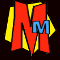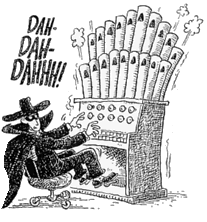#The TEN DIGIT Teaser ANSWER

## SOLUTION

 When does a number divide by 1-10? 1/ Anything divides by 1 2/ Number must be even (ending 2,4,6,8,0) 3/ Add the digits in the number. If the answer divides by 3, so does the number. 4/ The last two digits must divide by 4. To test: if the second-last digit is odd, the last digit must be 2 or 6. If the second-last digit is even, the last digit must be 0,4 or 8. 5/ Last digit must be 0 or 5. 6/ Number must be even and divide by 3. 7/ Remove last digit, and multiply it by 2. Subtract from other digits. Answer must be 0 or divide by 7. E.g. 623: remove 3 and x2 = 6. 62-6 = 56 which divides by 7, so does 623. 8/ The last three digits must divide by 8. To test: If the third last digit is even, the last two digits must divide by 8. If the third-last last digit is odd, last two digits must divide by 4, but NOT 8. 9/ Add the digits in the number. If the answer divides by 9, so does the number. 10/ There's a 0 on the end.

Call the number abcdefghij.

As the whole 10-digit number divides by 10, j = 0.
abcde must divide by 5. We have already used 0, therefore e = 5.

ab, abcd, abcd5f and abcd5fgh must all divide by 2. We have already used 0, so b, d, f and h must be 2, 4, 6 and 8 in some order.

By elimination, a, c, g and i must be 1, 3, 7 and 9 in some order.

abcd must be divisible by 4, and c must be odd; therefore d is 2 or 6.

Similarly h is also 2 or 6, so b and f must be 4 and 8 (in some order).

So far we have:

a = 1, 3, 7 or 9
b = 4 or 8
c = 1, 3, 7 or 9
d = 2 or 6
e = 5
f = 4 or 8
g = 1, 3, 7 or 9
h = 2 or 6
i = 1, 3, 7 or 9
j = 0

abc and abcdef must both divide by 3, so a + b + c and a + b + c + d + e + f must both divide by 3, and it follows that d + e + f must also divide by 3. Since d = 2 or 6, e = 5 and f = 4 or 8, the only possible combinations for these three digits are 258 or 654. Once d and f have been determined, b and h follow as there is only one possible digit remaining for each.

The two possibilities are:

a4c258g6i0

a8c654g2i0

For a4c258g6i0, a4c must be divisible by 3 and a, c must be some two of 1, 3, 7, 9. The only possibilities are 147 or 741. Furthermore, a4c258g6 must be divisible by 8, so 8g6 must be divisible by 8 which means g is either 1 or 9. This gives the following numbers as candidates: 1472589630 7412589630 For a8c654g2i0, a8c must be divisible by 3 and a, c must be two of 1, 3, 7, 9. The possibilities are 183, 189, 381, 387, 783, 789, 981 or 987. Furthermore, a8c654g2 must be divisible by 8, so 4g2 must be divisible by 8 and g is either 3 or 7. This eliminates 387 and 783 as possibilities for abc, leaving the following candidates:

1836547290

1896543270

1896547230

3816547290

7896543210

9816543270

9816547230

9876543210

 3/1 = 3 38/2 = 19 381/3 = 127 3816/4 = 954 38165/5 = 7633 381654/6 = 63609 3816547/7 = 545221 38165472/8 = 4770684 381654729/9 = 42406081 3816547290/10 = 381654729
All the eight numbers above satisfy all the divisions from 1-6 and 8-10. All that's left to do is to see if any of them have their first seven digits dividing by 7.

It turns out that the only set of first seven digits that works is 3816547, so the only correct answer to the question is ...drum roll...3816547290

With big thanks to MICHAEL JONES for explaining how this all works!To the Teacher Page